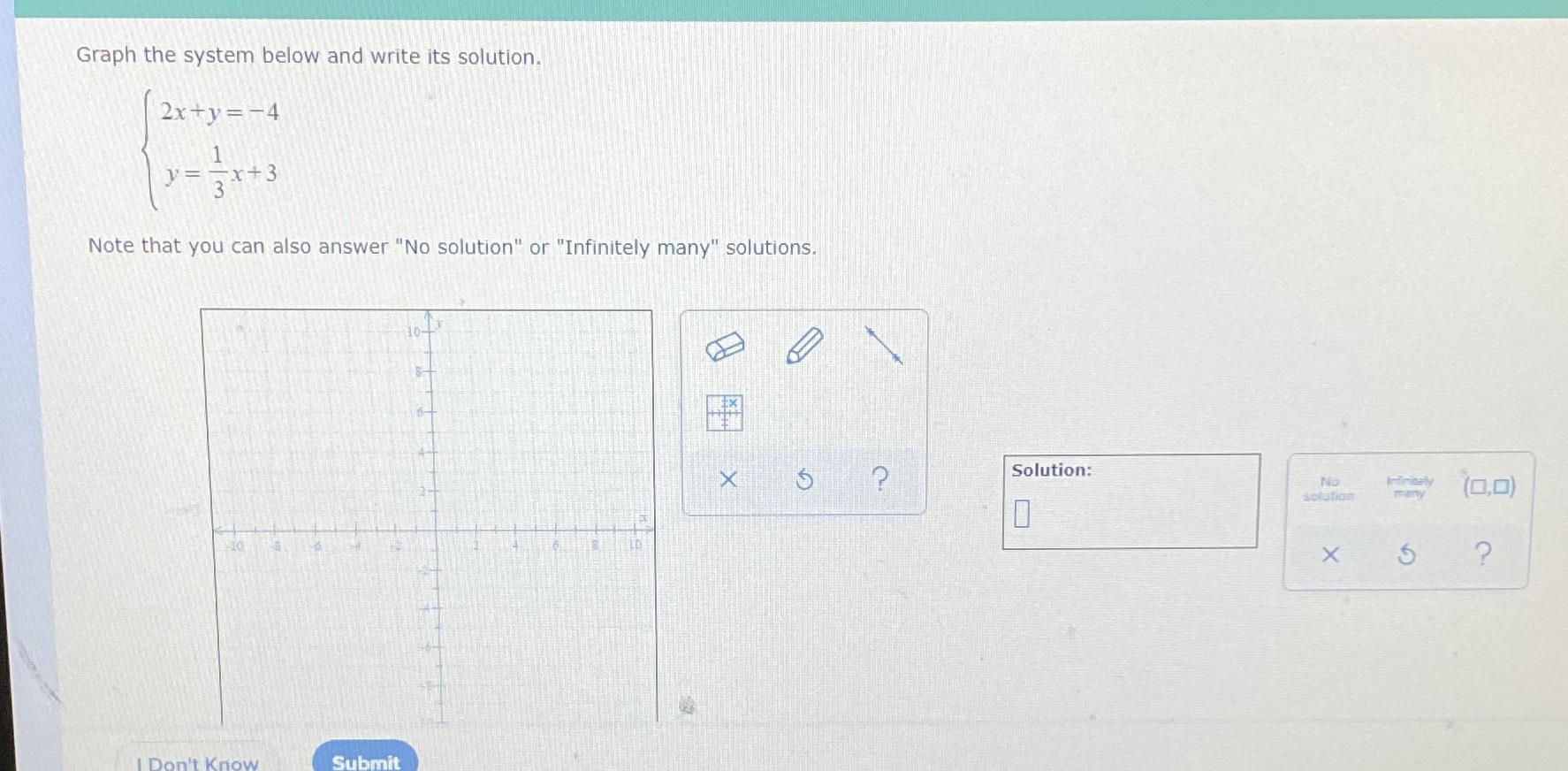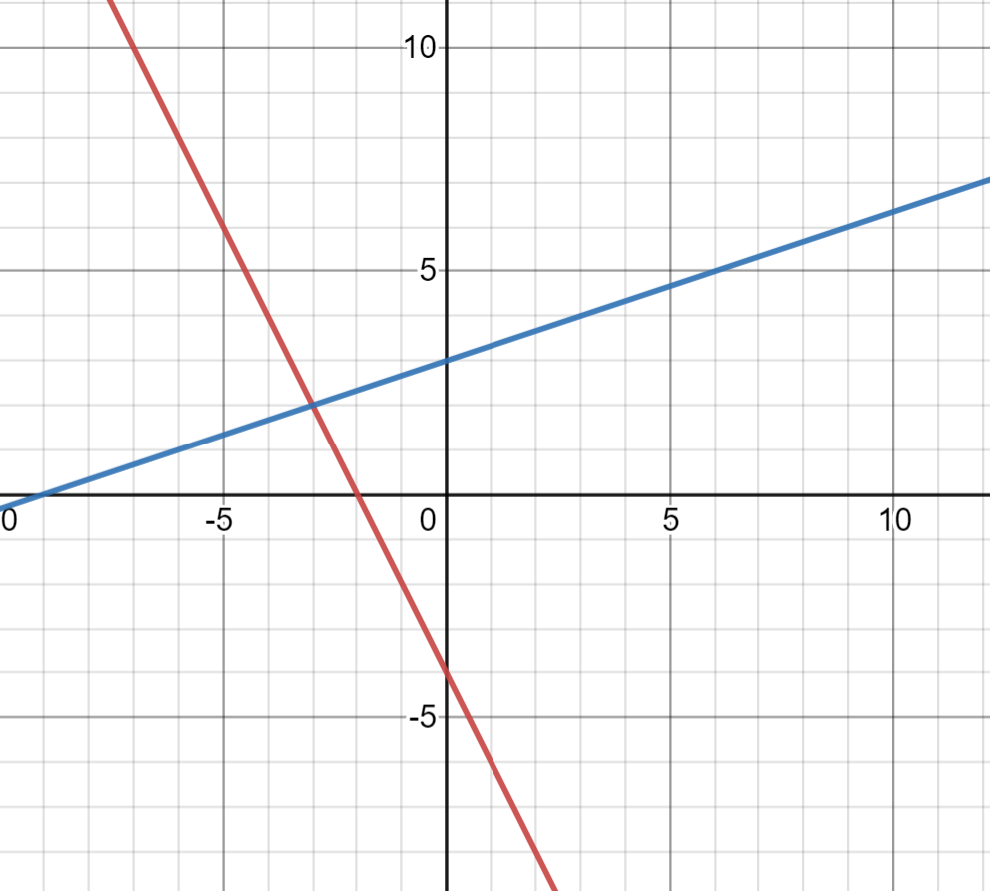### ¿Todavía tienes preguntas de matemáticas?

Pregunte a nuestros tutores expertos
Algebra
PreguntaGraph the system below and write its solution. $$\left\{ \begin{array} { l } { 2 x + y = - 4 } \\ { y = \frac { 1 } { 3 } x + 3 } \end{array} \right.$$Note that you can also answer "No solution" or "Infinitely many" solutions.